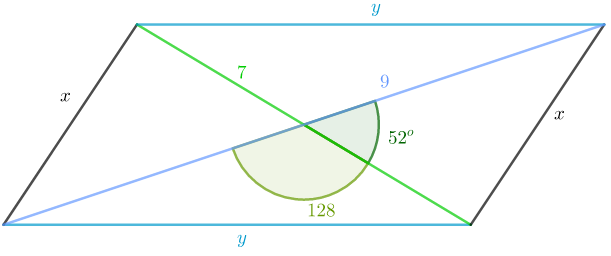### Math Notes

Subjects

#### Trigonometry Solutions

##### Topics || Problems

The diagonals of a parallelogram are 7 inches and 9 inches respectively, they intersect at an angle of $$52^o$$. Find the sides of the parallelogram.The intesection of the diagonals of a parallelogra bisect each diagonals. Thus, the values of $$x ~ and ~y$$ can be calculated using cosine law.

Solve the value of $$x:$$$$x^2 = 3.5^2 + 4.5^2 - 2(3.5)(2.5) \cos 52$$

$$x = 3.62 ~inches$$

Solve $$y:$$

$$y^2 = 3.5^2 + 4.5^2 -2(3.5)(4.5) \cos 128$$

$$y = 7.20 ~inches$$